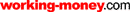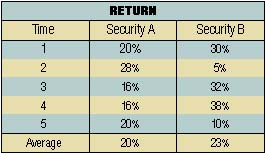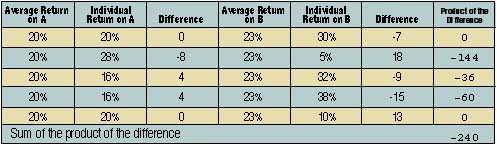HOT TOPICS LIST

LIST OF TOPICS

08/29/01 11:45:51 AM PST
by Justin Sorci

Save yourself! To protect your portfolio from market volatility, create a diversified portfolio by using the oppressively named correlation coefficient, but it's not as complicated as it sounds.

Does your portfolio include only tech stocks? It may have worked in your favor during the 1999 to early 2000 bull rally, but how about after the fall of the tech sector? If you're a typical investor, your entire portfolio probably suffered. It was probably at that point that you realized hindsight being 20/20, after all that you might have been better off if you had a portfolio made up of stocks that didn't all move in the same direction at the same time. One way to create the diversified portfolio you need is to use correlation coefficients. The term may conjure nightmarish memories of that statistics class you dreaded in college, but it's not that complicated. Simply, the correlation coefficient measures the strength of the relationship between two securities. Here's how you can calculate and use it.1: Although returns on both are positive, sometimes one outperformed the other.

### CALCULATION

Your first step will be to determine the average return for each security you plan to add to your portfolio. Consider the returns of the two securities in Figure 1. Both yield positive returns over this particular time frame. Sometimes security A outperforms security B and vice versa. Next, calculate the variability between the two securities. The covariance measures relative variability of returns between security A and security B. The covariance is the sum of the product of the differences between each security's return and its average, divided by the number of observations (time frame) minus 1.

2: Sum of the product of the differences is a necessary component in calculating covariance

Covariance = S product of the differences

(no. of observations-1)

When

S = Don't be alarmed! This Greek letter,

sigma, just means "total" like the "=" sign

Covariance = -240/(5-1) = -60

The results of the calculation of covariance between security A and security B can be seen in Figure 2.A positive covariance indicates that the two securities move in the same direction. A negative covariance indicates that the two securities move in opposite directions, and thus having both in your portfolio would help you in your desire to diversify. In addition, larger numbers have stronger relationships, while smaller numbers have weaker relationships. The last step is to convert the covariance into the correlation coefficient:

Correlation coefficient =

Covariance

AB

(Standard deviation of A)(Standard dev. of B)

Standard deviation measures the difference between the stock's return and its average return. It's the square root of the stock's variance.

The values for the correlation coefficient range from +1 to -1. A value equal to 1 indicates that the two securities move in unison (that is, perfect positive correlation). A value equal to -1 indicates that the two securities move exactly opposite of each other. A value equal to zero indicates that the returns on the two securities have no relationship.

Correlation coefficient =

-60/((4.8989)(14.5602)) = -0.84117

A correlation coefficient of -0.84117 indicates a fairly strong negative relationship between security A and security B that is, the two securities tend to move in opposite directions (also known as an inverse relationship). The correlation coefficient can be taken a step further by squaring the answer: (0.84117)(0.84117) = 0.7075. This value, known as a coefficient of determination, indicates that 70.75% of the variation in security A can be explained by the variation in security B and vice versa.

### SUMMARY

Diversifying our portfolios is key to making sure our investments stay healthy. Using correlation coefficients can help us make sure that even if some of the stocks in our portfolios suffer dramatic declines, we have others that will either stand pat or even climb in value.

To best protect your investments, experiment with different combinations of securities and determine their relationships. An ideal portfolio should consist of securities that are not highly correlated to each other. Instead of having a portfolio that consists only of technology stocks, you should end up with one that covers various sectors and will protect you from severe downfalls. You may be surprised at the results.

Justin Sorci has been trading stocks and commodities since 1986.

### REFERENCES

Mayo, Herbert B. . Investments: An Introduction, fifth ed., The Dryden Press.

Justin Sorci

 Comments or Questions? Article Usefulness 5 (most useful) 4 3 2 1 (least useful)